# Detecting regions of Neanderthal ancestry with Deep LearningDetecting regions of Neanderthal ancestry with Deep Learning.I demonstrated how to use Deep Learning for Ancient DNA, Single Cell Biology OMICs Data Integration,

In the previous posts, I demonstrated how to use Deep Learning for Ancient DNA, Single Cell Biology OMICs Data Integration, Clinical Diagnostics and Microscopy Imaging Today we are going to dive into the exciting History of Human Evolution and learn that it is straightforward to borrow methodology from the Natural Language Processing (NLP) and apply it to Human Population Genetics in order to infer regions of [Neanderthal introgression]

## Brief History: Out of Africa

When ancestors of Modern Humans migrated out of Africa ~ 50 000 - 70 000 years ago, they encountered Neanderthals and Denisovans, two groups of ancient hominins that populated Europe and Asia at that time. We know that Modern Humans interbred with both Neanderthals and Denisovans since there is evidence of the presence of their DNA in genomes of Modern Humans of non-African origin. These great genetic advances in the History of Human Evolution became possible due to the sequencing of Neanderthal and Denisovan genomes in 2010 and 2012, respectively, by the group of Svante Pääbo.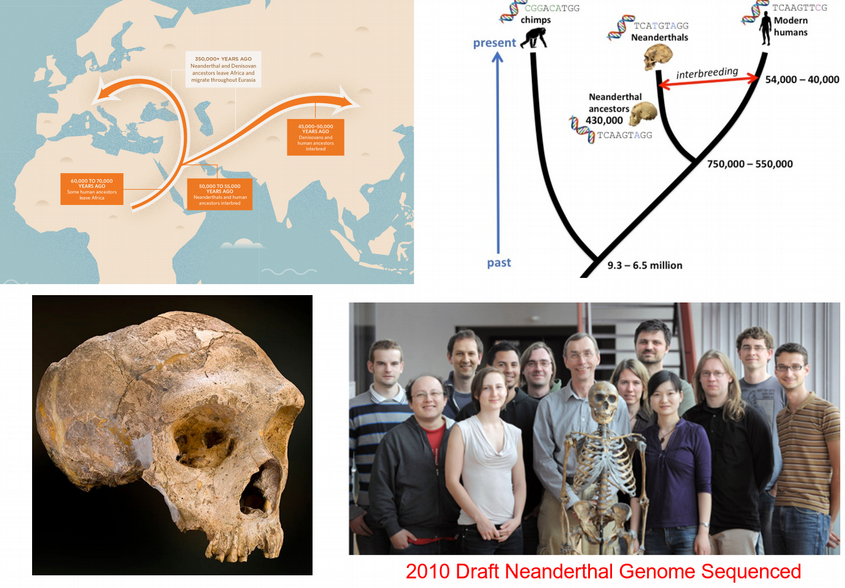There were a few attempts made to infer the exact positions of DNA segments inherited from Neanderthals and Denisovans. They utilized various genomics resources such as 1000 Genomes project and computed different measures of local similarity of a non-African genome toward the high-quality Neanderthal genome and divergence with respect to African genomes (right figure below).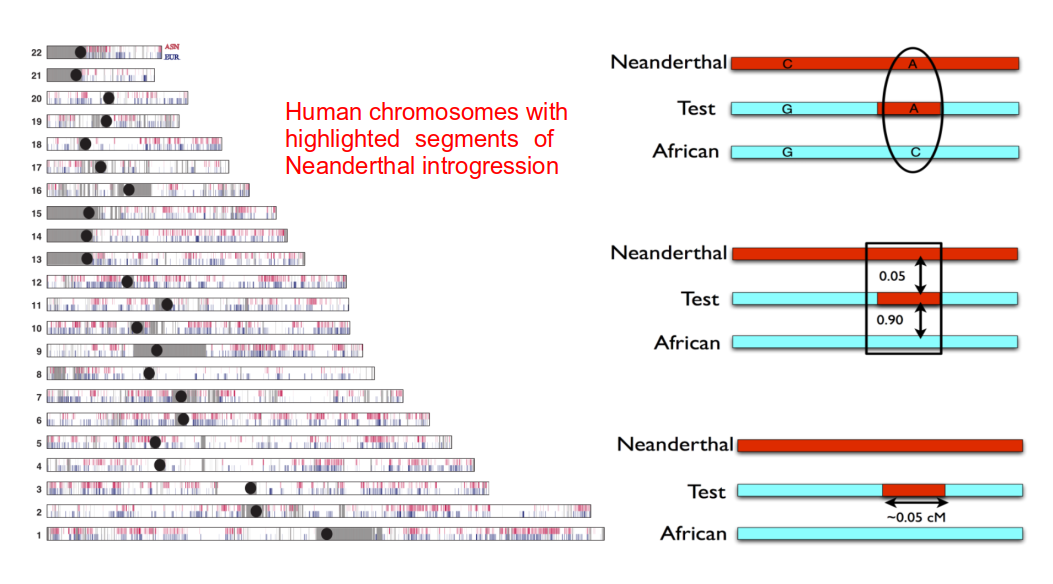S* / S’ statistics and Conditional Random Field (CRF) were applied to detect Neanderthal introgression

Those similarity measures (summary statistics) despite being interpretable and efficient result in the loss of information because they attempt to capture a stretch of DNA in a single number without considering the ordered sequence of nucleotides itself. Other attempts to scan Neanderthal introgression across a genome use Hidden Markov Model (HMM) which is a memoryless model that again does not account for long-range correlations between nucleotides along the DNA sequence. This is where the power of Deep Learning to process raw genomic sequences and utilize the long memory of nucleotide linkage across the genome with RNNs / LSTMs and CNNs can be incredibly beneficial.

# Potential of Deep Learning for Ancient Genomics

Currently, Deep Learning is basically ignored in Evolutionary Biology despite its great potential for working with Genomics data that represent one of the major data sources in the present Evolutionary Science and Ancient DNA research areas. Recent attempts utilize simulated genomic data despite the availability of real Genomics data that often have annotated regions with well understood biological function. Deep Learning is ideally suited for working with Genomics and Ancient Genomics simply because one genome is actually a Big Data. To realize this, just think about chopping the 310⁹ nucleotides long genome into stretches of 1000 nucleotides which brings 310⁶ training examples to be used for Deep Learning providing that an annotation (labels) can be figured out for each of the DNA stretches. That is a massive amount of training data!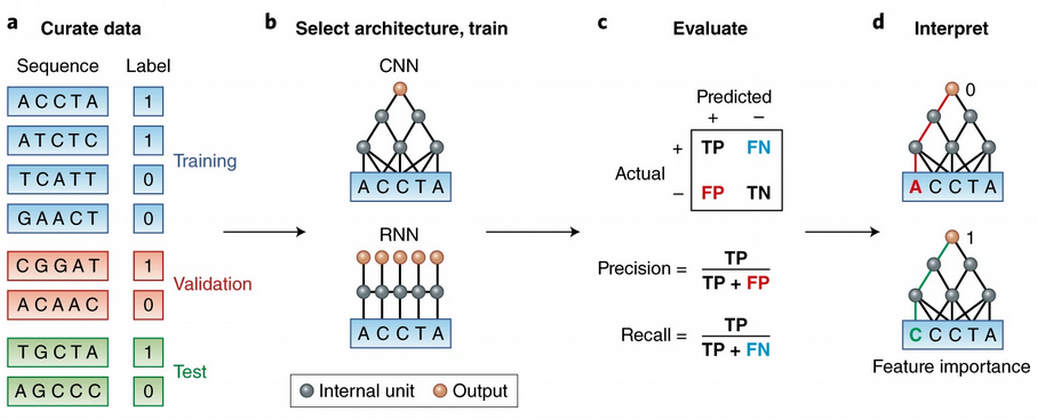Deep Learning for Genomics from Zou et al. Nature Genetics 51, pages12–18 (2019)

I demonstrated how to use this idea for discriminating ancient from modern DNA sequences in my previous post. There I used a 1D Convolutional Neural Network (CNN) and a one-hot-encoded representation of the sequences. Here I am going to demonstrate another way to represent DNA sequences for input into Deep Learning. Please look at the left figure below, this is what we usually mean by text. However, this is not what people in Genomics mean by text. What they mean by text is shown to the right. DNA sequence is a text! It looks boring but with time you start seeing things in strings. What if I told you that I see a gene there? What if I told you that I see a gene strongly linked to Type 2 Diabetes (T2D) and we likely inherited this gene from Neanderthals?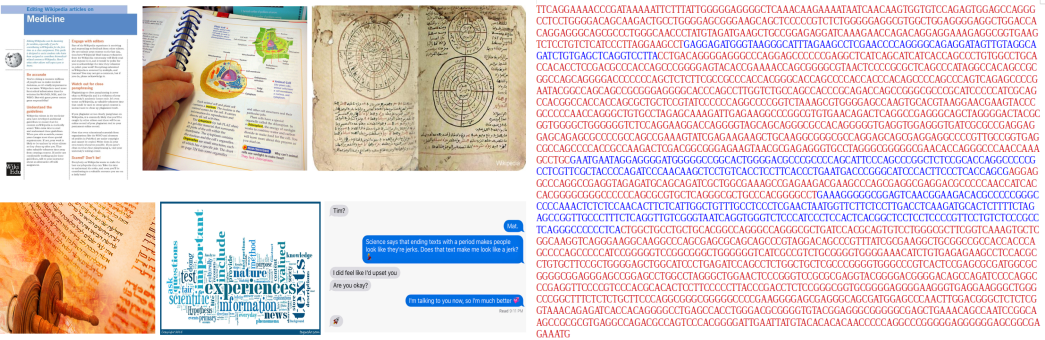What people usually mean by text (left), vs. what bioinformaticians mean by text (right), SLC16A11 gene

Now, if DNA sequence is a text we can apply all the apparatus from the Natural Language Processing (NLP) to such texts. However, where are sentences in the DNA texts and where are words? If we were to predict whether a bunch of DNA sequences (the bunch can be considered as a text) was inherited from Neanderthals or not, one DNA sequence from the bunch can be considered as a sentence of the text, and a k-mer (sub-sequence) can be treated as a word.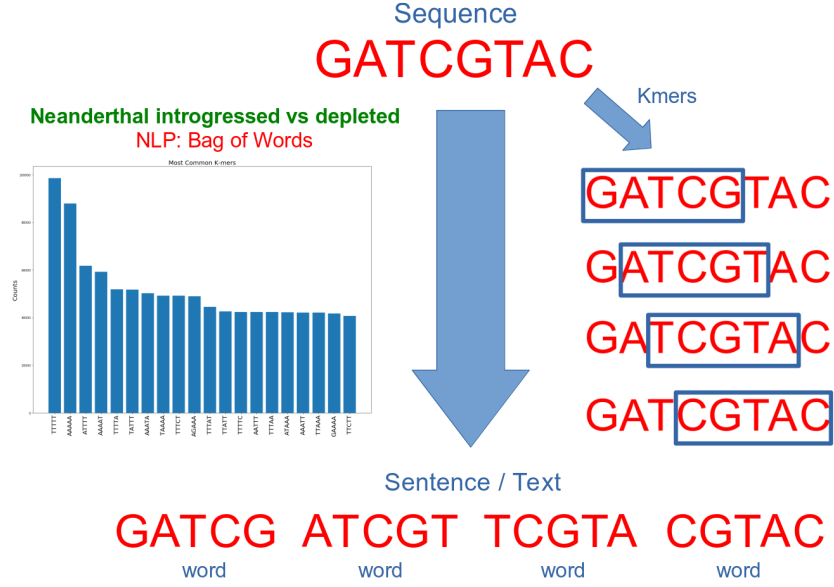DNA sequence is a sentence that can be split into k-mers, space-delimited k-mers can be seen as words

Once we converted DNA sequences into space-delimited k-mers / words, we are done. Now we can turn to advanced NLP techniques and use e.g. a simple Bag Of Words model for comparing frequencies of words / k-mers between the sequences inherited from Neanderthals and sequences of depleted archaic ancestry.

# Prepare Sequences for Sentiment Analysis

Now let us demonstrate how to practically use Machine / Deep Learning and Natural Language Processing (NLP) for identifying the regions of Neanderthal introgression in modern human genomes. Here I will formulate the following problem to solve:

### Show me a stretch of your DNA and I will predict how likely it was inherited from Neanderthals

As a training data set we are going to use coordinates of the candidate regions for Neanderthal introgression from Vernot and Akey, Science 2016 identified using S-statistic on Europeans and Asians from the 1000 Genomes Project, the data can be downloaded from here https://drive.google.com/drive/folders/0B9Pc7_zItMCVWUp6bWtXc2xJVkk. We used the introgressed haplotypes file LL.callsetEUR.mr_0.99.neand_calls_by_hap.bed.merged.by_chr.bed and selected only unique coordinates, so we ended up with *83 601 regions of Neanderthal ancestry in modern Europeans. Let us read the coordinates and have a look at the length distribution of the Neanderthal introgressed regions.

IntrLengthDistr.py

``````import pandas as pd
import seaborn as sns
import matplotlib.pyplot as plt
intr_lengths = intr_coords.iloc[:, 2]-intr_coords.iloc[:, 1]
sns.distplot(intr_lengths)
plt.title("Distribution of Lengths of Neandertal Introgressed Regions", fontsize = 20)
plt.xlabel("Lengths of Neandertal Introgressed Regions", fontsize = 20)
plt.ylabel("Frequency", fontsize = 20)``````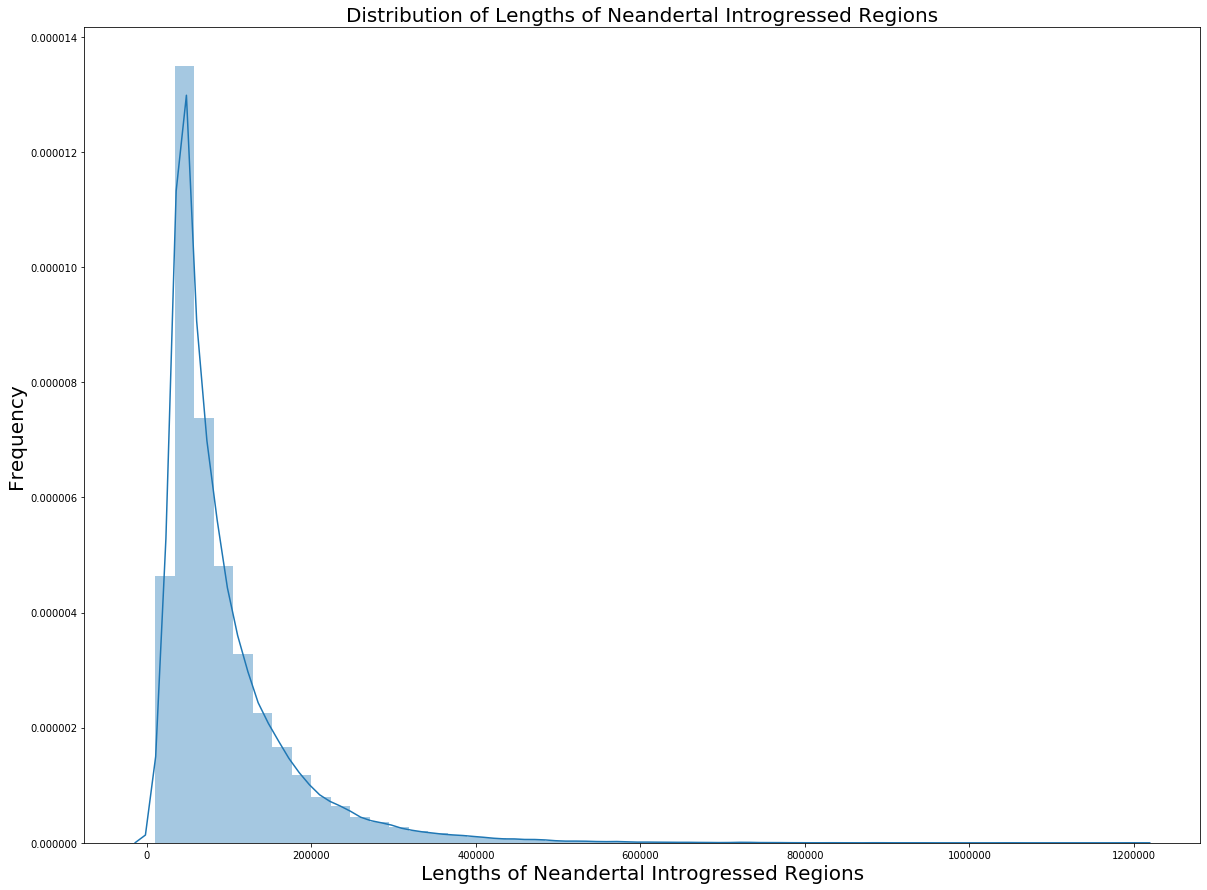We can see that the length of Neanderthal introgression segments varies from 10 kbp to 1.2 Mbp with the mean of ~100 kbp. Now we are going to use those coordinates and extract the actual sequences from the hg19 version of the human reference genome which has a fasta-file format and can be downloaded from http://hgdownload.cse.ucsc.edu/goldenPath/hg19/bigZips/. We can of course use Python for sequence extraction but it is much faster to do it with the C++ based samtools.

build_intr.py

``````import subprocess
a = 0
with open('hg19_intr_regions.fa', 'a') as fp:
for i in range(intr_coords.shape):
coord = str(str(intr_coords.iloc[i, 0]) + ':'
+ str(intr_coords.iloc[i, 1]) + '-' + str(intr_coords.iloc[i, 2]))
subprocess.run(['samtools', 'faidx', 'hg19.fa.gz', str(coord)], stdout = fp)
a = a + 1
if a%10000 == 0:
print('Finished ' + str(a) + ' Neanderthal introgressed haplotypes')``````

Now we are going to build a Pandas DataFrame with coordinates of segments outside of the Neanderthal introgression coordinates. For this purpose, we are going to randomly draw DNA stretches of the same length as the introgression regions on the same chromosome and check whether these stretches intersect with any of the introgressed regions. In this way, we construct two sets of non-overlapping coordinates: introgressed and depleted regions.

build_depl.py

``````import numpy as np
chr_sizes = chr_sizes.drop([2, 3, 4], axis = 1)
chr_list = []; start_list = []; end_list = []
intr_lengths = list(intr_coords.iloc[:, 2] - intr_coords.iloc[:, 1])
a = 0
for i in range(intr_coords.shape):
chr_df = intr_coords[intr_coords.isin([intr_coords.iloc[i,0]])]
overlap = True
while overlap == True:
reg_start = np.random.randint(1, int(chr_sizes[chr_sizes ==
intr_coords.iloc[i,0]].iloc[:,1]))
reg_end = reg_start + intr_lengths[i]
for j in range(chr_df.shape):
b1 = chr_df.iloc[j,1]
b2 = chr_df.iloc[j,2]
if (reg_start > b1 and reg_start < b2) or (reg_end > b1 and reg_end < b2) or \
(b1 > reg_start and b1 < reg_end) or (b2 > reg_start and b2 < reg_end):
overlap = True
break
else:
overlap = False
chr_list.append(intr_coords.iloc[i,0])
start_list.append(reg_start)
end_list.append(reg_end)
a = a + 1
if a%10000 == 0:
print('Finished ' + str(a) + ' Neanderthal introgressed haplotypes')
depl_coords = pd.DataFrame({'0': chr_list, '1': start_list, '2': end_list})
depl_coords.to_csv("Akey_depl_coords.bed", index = False, header = False, sep = "\t")``````

Once the DataFrame containing regions of depleted Neanderthal ancestry has been built, we can again extract the actual DNA sequences from the hg19 fasta corresponding to the depleted segments with samtools, here I skip for brevity this step but check the complete Jupyter notebook on github to see the details. Taking a closer look at the extracted introgressed and depleted sequences we can notice quite a few N-nucleotide containing sequences. This is the missing data in Genomics, i.e. positions not resolved by the sequencing technologies. In order not to let the missing data affect our analysis I omitted the sequences containing at least one N-nucleotide, this can be efficiently done in BioPython:

clean_N.py

``````from Bio import SeqIO
intr_file = 'hg19_intr_regions.fa'
depl_file = 'hg19_depl_regions.fa'
a = 0; i = 0
with open('hg19_intr_clean.fa', 'a') as intr_out,open('hg19_depl_clean.fa', 'a') as depl_out:
for intr, depl in zip(SeqIO.parse(intr_file, 'fasta'), SeqIO.parse(depl_file, 'fasta')):
upper_intr = intr.seq.upper()
upper_depl = depl.seq.upper()
a = a + 1
if a%10000 == 0:
print('Finished ' + str(a) + ' entries')
if 'N' not in str(upper_intr) and 'N' not in str(upper_depl):
intr.seq = upper_intr
SeqIO.write(intr, intr_out, 'fasta')
depl.seq = upper_depl
SeqIO.write(depl, depl_out, 'fasta')
i = i + 1
else:
continue
print('We have processed ' + str(a) + ' entries and written ' + str(i)
+ ' entries to two fasta-files')``````

Now the data is prepared for inputting into NLP analysis. Let us proceed with the simple Bag Of Words model, i.e. looking at the difference in frequency of k-mers between Neanderthal introgressed vs. depleted sequences.

## Sentiment Analysis: Introgressed vs. Depleted

We are going to start with formatting the sequences from the two fasta-files as texts with space-delimited k-mers as words. Because of memory limitations of my laptop I read only first 10,000 nucleotides from each sequence, then I used the function getKmers that splits each sequence into k-mers and merged the k-mers in space-delimited manner, so at the end, I had a list of sentences, each of them represents a list of words / k-mers.

build_texts.py

``````from Bio import SeqIO
from Bio.Seq import Seq
intr_file = 'hg19_intr_clean.fa'; depl_file = 'hg19_depl_clean.fa'; e = 0
intr_seqs = []; depl_seqs = []
for intr, depl in zip(SeqIO.parse(intr_file, 'fasta'), SeqIO.parse(depl_file, 'fasta')):
cutoff = 10000
my_intr_seq = str(intr.seq)[0:cutoff]
my_depl_seq = str(depl.seq)[0:cutoff]
intr_seqs.append(my_intr_seq)
depl_seqs.append(my_depl_seq)
e = e + 1
if e%20000 == 0:
print('Finished ' + str(e) + ' entries')

def getKmers(sequence, size):
return [sequence[x:x+size].upper() for x in range(len(sequence) - size + 1)]

kmer = 5
intr_texts = [' '.join(getKmers(i, kmer)) for i in intr_seqs]
depl_texts = [' '.join(getKmers(i, kmer)) for i in depl_seqs]``````

Now we can easily visualize k-mer frequencies in Neanderthal introgressed and depleted sequences using the Counter class in Python for efficient word counting.

visualize_kmer_counts.py

``````from collections import Counter
import matplotlib.pyplot as plt
fig = plt.figure(figsize = (20,18))
fig.subplots_adjust(hspace = 0.4, wspace = 0.4)

plt.subplot(2, 1, 1)
intr_sentences = [item.split(' ') for item in intr_texts]
D = dict(Counter([item for sublist in intr_sentences for item in sublist]).most_common(20))
plt.bar(range(len(D)), list(D.values()), align='center')
plt.title('Most Common K-mers for Neanderthal Introgressed Regions', fontsize = 20)
plt.ylabel("Counts", fontsize = 20); plt.xticks(rotation = 90)
plt.xticks(range(len(D)), list(D.keys()), fontsize = 20)

plt.subplot(2, 1, 2)
depl_sentences = [item.split(' ') for item in depl_texts]
D = dict(Counter([item for sublist in depl_sentences for item in sublist]).most_common(20))
plt.bar(range(len(D)), list(D.values()), align='center')
plt.title('Most Common K-mers for Neanderthal Depleted Regions', fontsize = 20)
plt.ylabel("Counts", fontsize = 20); plt.xticks(rotation = 90)
plt.xticks(range(len(D)), list(D.keys()), fontsize = 20)``````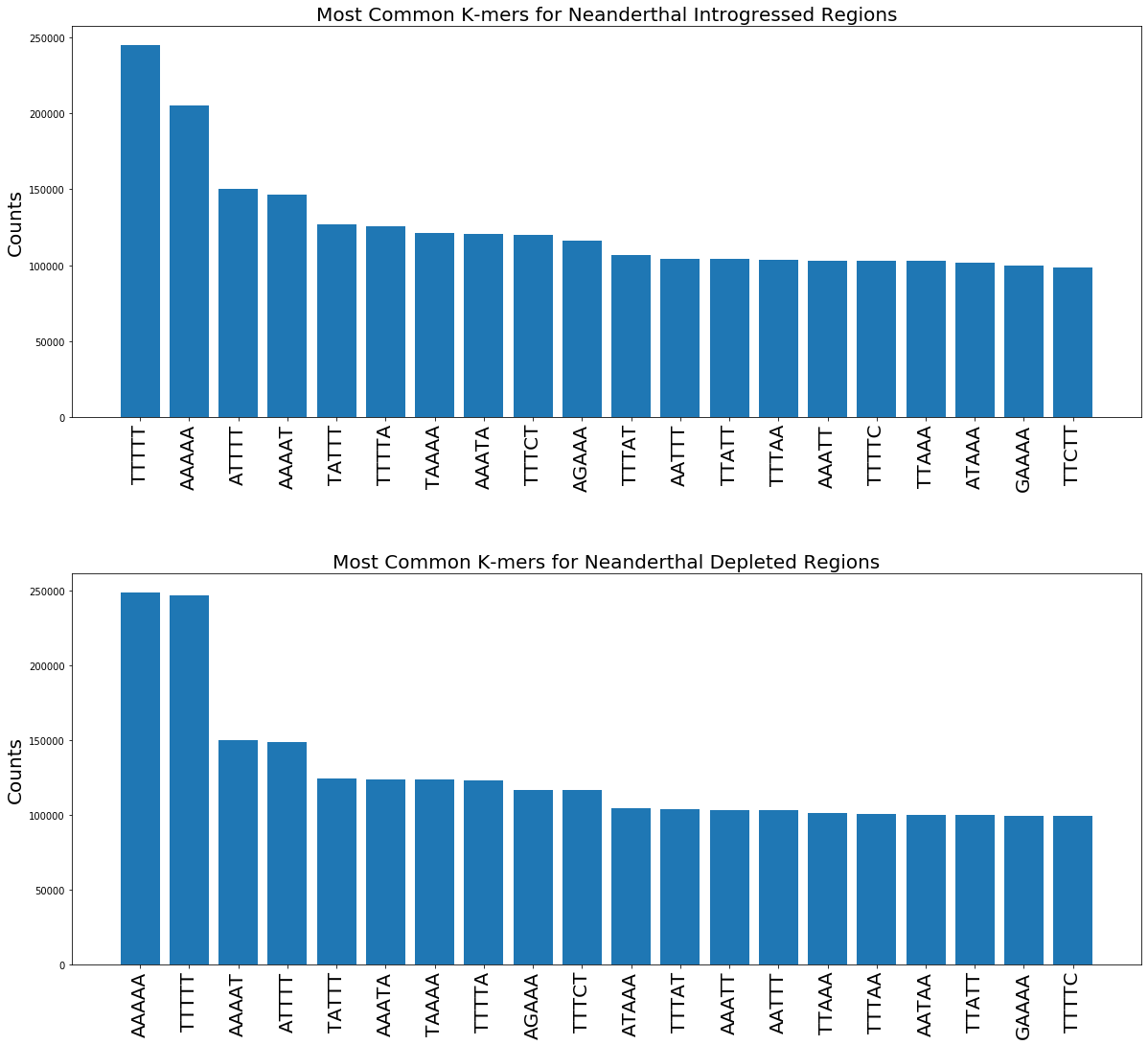Although A- and T-rich k-mers seem to be most common for both Neanderthal introgressed and depleted regions, we observe small differences in the k-mer counts between the two cases which can be indicative for non-identical k-mer composition in the introgressed and depleted sequences. Next, we encode the words / k-mers as integers with CountVectorizer class which simply builds a vocabulary of the text (number of unique k-mers) and counts occurrences of each word / k-mer in each sentence/sequence.

count_vect.py

``````import numpy as np
from sklearn.model_selection import train_test_split
from sklearn.feature_extraction.text import CountVectorizer
merge_texts = intr_texts + depl_texts
labels = list(np.ones(len(intr_texts))) + list(np.zeros(len(depl_texts)))
cv = CountVectorizer()
X = cv.fit_transform(merge_texts)
X = np.int32(X.toarray())
X_train, X_test, y_train, y_test = train_test_split(X, labels,
test_size = 0.20, random_state = 42)``````

After we have split the data set into train and test subsets, we define a feed-forward neural network with Stochastic Gradient Descent (SGD) optimizer + Momentum and L1 weight regularizer.

mlp.py

``````from keras.models import Sequential
from keras.regularizers import l2, l1
from keras.callbacks import ModelCheckpoint
from keras.layers import Dense, Flatten, Dropout

model = Sequential()
model.add(Dense(3000, input_shape = (X.shape, ), activation = 'sigmoid',
kernel_regularizer = l1(0.00001)))
sgd = SGD(lr = 0.0001, momentum = 0.9, nesterov = False)
model.compile(loss = 'binary_crossentropy', optimizer = sgd, metrics = ['binary_accuracy'])
checkpoint = ModelCheckpoint("weights.best.hdf5", monitor='val_binary_accuracy', verbose=1,
save_best_only = True, mode = 'max')
history = model.fit(X_train, y_train,
epochs = 200, verbose = 1, validation_split = 0.2, batch_size = 32,
shuffle = True, callbacks = [checkpoint])``````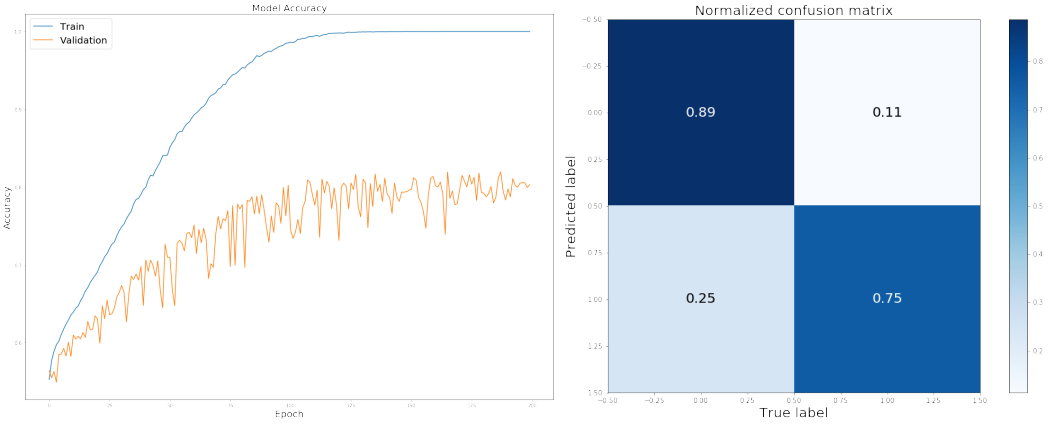MLP Accuracy training curve (left) and confusion matrix of evaluation on test data set (right)

The model reached the accuracy of 82.2% on the test data set classifying the sequences of Neanderthal introgressed vs. depleted origin. This was a clearly better result compared to linear models such as Logistic Regression, Support Vector Machines (SVM) or Naive Bayes Classifier, however, unexpectedly, the Random Forest Classifier reached even higher accuracy of 84.5% on the same test data set. Displaying the feature importances of the Random Forest model we observe such k-mers as AAAAA, CAAAA, CATTT and TTTTT to be the most predictive. We immediately get an intuition that the prediction of Neanderthal introgressed/depleted regions has something to do with the GC/AT content because the most predictive k-mers are extremely AT-rich.

RF.py

``````import pickle
import matplotlib.pyplot as plt
from sklearn.ensemble import RandomForestClassifier

classifier = RandomForestClassifier(n_estimators = 500)
classifier.fit(X_train, y_train)
y_pred = classifier.predict(X_test)
pickle.dump(classifier, open('RF_model_Neand_Intr_vs_Depl.sav', 'wb'))

importances = classifier.feature_importances_
std = np.std([tree.feature_importances_ for tree in classifier.estimators_], axis = 0)
indices = np.argsort(importances)[::-1]
plt.title("Feature importances", fontsize = 20)
plt.bar(range(X_train.shape)[0:50], importances[indices][0:50],
yerr = std[indices][0:50], align = "center")
plt.xticks(rotation = 90); plt.ylabel('Score', fontsize = 20)
plt.xticks(range(X_train.shape)[0:50], np.array(names)[indices][0:50])``````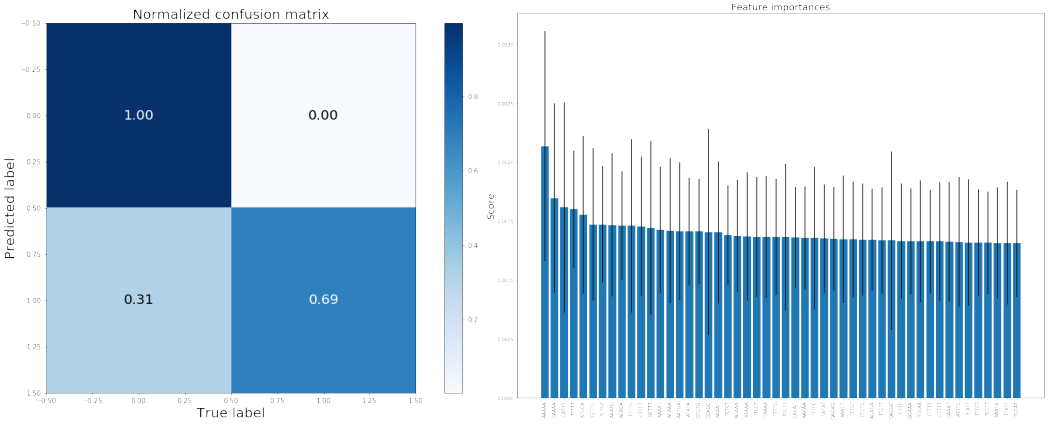Random Forest classifier confusion matrix (left) and feature importances dominated by AT-rich k-mers (right)

From the Confusion Matrix for Random Forest Classifier (above) it becomes clear that the model had a very high accuracy when predicting segments of depleted Neanderthal ancestry, however, performed very poorly (worse than the neural network) on classifying introgressed regions.

## Predict Genes Inherited from Neanderthals

Now let us come back to the question of predicting from a given DNA sequence whether it was inherited from Neanderthals or not. Here I will generate such predictions for human protein-coding genes. Let us download the RefSeq gene annotation file for hg19 here http://genome.ucsc.edu/cgi-bin/hgTables, clean it and use the coordinates of the genes in order to extract their sequences from the reference genome fasta-files similarly to how we did it for the introgressed and depleted regions, see details in the Jupyter notebook on github. Again we construct the gene texts via space-delimited concatenation of the k-mers.

build_gene_texts.py

``````from Bio import SeqIO
gene_seqs = []; gene_ids = [], a = 0
for gene in SeqIO.parse('hg19_gene_clean.fa', 'fasta'):
cut = 8800
if len(str(gene.seq)) < cut:
continue
s_gene = str(gene.seq)[0:cut]
if s_gene.count('A')>0 and s_gene.count('C')>0 and s_gene.count('G')>0 \
and s_gene.count('T')>0:
gene_seqs.append(s_gene)
gene_ids.append(str(gene.id))
a = a + 1
if a%10000 == 0:
print('Finished ' + str(a) + ' genes')

def getKmers(sequence, size):
return [sequence[x:x+size].upper() for x in range(len(sequence) - size + 1)]

kmer = 5
gene_texts = [' '.join(getKmers(i, kmer)) for i in gene_seqs]``````

Next, we are going to use the trained Random Forest classifier to generate predictions for the sequences of the genes that were previously converted to texts. Hence, the gene texts are converted to integers with CountVectorizer.

gene_predict.py

``````import pickle
import pandas as pd
from sklearn.feature_extraction.text import CountVectorizer

cv = CountVectorizer(); X_gene = cv.fit_transform(gene_texts)
gene_predictions = classifier.predict(X_gene.toarray())
gene_predictions_prob = classifier.predict_proba(X_gene.toarray())
gene_predictions_prob_0 = [i for i in gene_predictions_prob]
gene_predictions_prob_1 = [i for i in gene_predictions_prob]

gene_ids = []; gene_symbol = []
with open('gene_ids.txt','r') as fin:
for line in fin:
line = line.split('\t')
gene_ids.append(line)
gene_symbol.append(line.rstrip())

gene_pred_df = pd.DataFrame({'Gene': gene_ids, 'Gene_Symbol': gene_symbol,
'Predict': gene_predictions,
'Prob_0': gene_predictions_prob_0,
'Prob_1': gene_predictions_prob_1})
gene_pred_df = gene_pred_df.sort_values(['Prob_1'], ascending = False)``````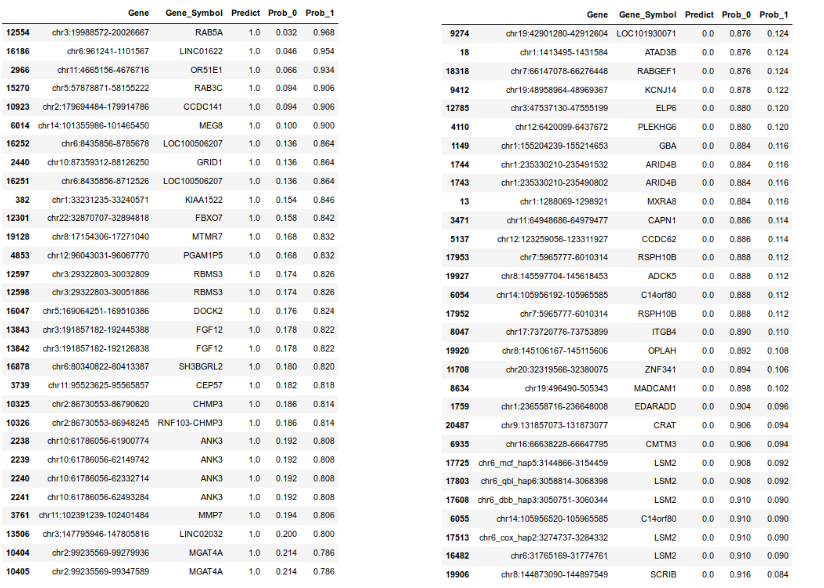Genes predicted to have high (left) and low (right) probability to be inherited from Neanderthals

In this way, for each gene we generate a probability (Prob_1) to be inherited from Neanderthals and to be a native human gene (Prob_0). We can easily display the genes with highest Prob_1 (lowest Prob_0) and highest Prob_0 (lowest Prob_1), please see the gene lists above. Remarkably, out of ~22000 protein-coding genes, only 477 were predicted to contain Neanderthal DNA. That is a very interesting observation, we will revisit it later.

## Visualization of K-mer / Word Embeddings

The vocabulary of the genomic texts produced by concatenating k-mers can be visualized via a Word Embedding model Word2Vec which makes intelligent guesses about the similarity between words (k-mers in our case). Once fit on our data Word2Vec represents each k-mer as a 100-dimensional latent vector, the vectors can be viewed as another numeric representation of our data that can be input into any dimension reduction technique for further exploration. Here we are going to embed and visualize with UMAP and tSNE the k-mers used for the Neanderthal introgression vs. depletion Sentiment Analysis. We can detect two obvious k-mer clusters. A closer look shows that one of them seems to be enriched for AT-rich k-mers and the other one (smaller one) is predominantly composed of GC-rich k-mers.

umap_word2vec.py

``````from umap import UMAP
import matplotlib.pyplot as plt
from gensim.models import Word2Vec

kmer = 5
sequences = intr_seqs + depl_seqs; sentences = []
for i in range(len(sequences)):
sentences.append(getKmers(sequences[i], kmer))

model = Word2Vec(sentences, min_count = 2, workers = 4)
X = model[model.wv.vocab]

X_reduced = PCA(n_components = 5).fit_transform(X)
umap_model = UMAP(n_neighbors = 30, min_dist = 0.2, n_components = 2)
umap = umap_model.fit_transform(X_reduced)
plt.scatter(umap[:, 0], umap[:, 1], s = 10, cmap = 'tab10')
plt.title('UMAP: All K-mers', fontsize = 20)
plt.xlabel("UMAP1", fontsize = 20); plt.ylabel("UMAP2", fontsize = 20)
words = list(model.wv.vocab)
for i, word in enumerate(words):
if word == 'AAAAA':
plt.text(umap[i, 0], umap[i, 1], word, fontsize = 30, c = 'green')
elif word == 'CAAAA':
plt.text(umap[i, 0], umap[i, 1], word, fontsize = 30, c = 'green')
elif word == 'CATTT':
plt.text(umap[i, 0], umap[i, 1], word, fontsize = 30, c = 'green')
elif word == 'TTTTT':
plt.text(umap[i, 0], umap[i, 1], word, fontsize = 30, c = 'green')
else:
plt.text(umap[i, 0], umap[i, 1], word, fontsize = 10, c = 'red')``````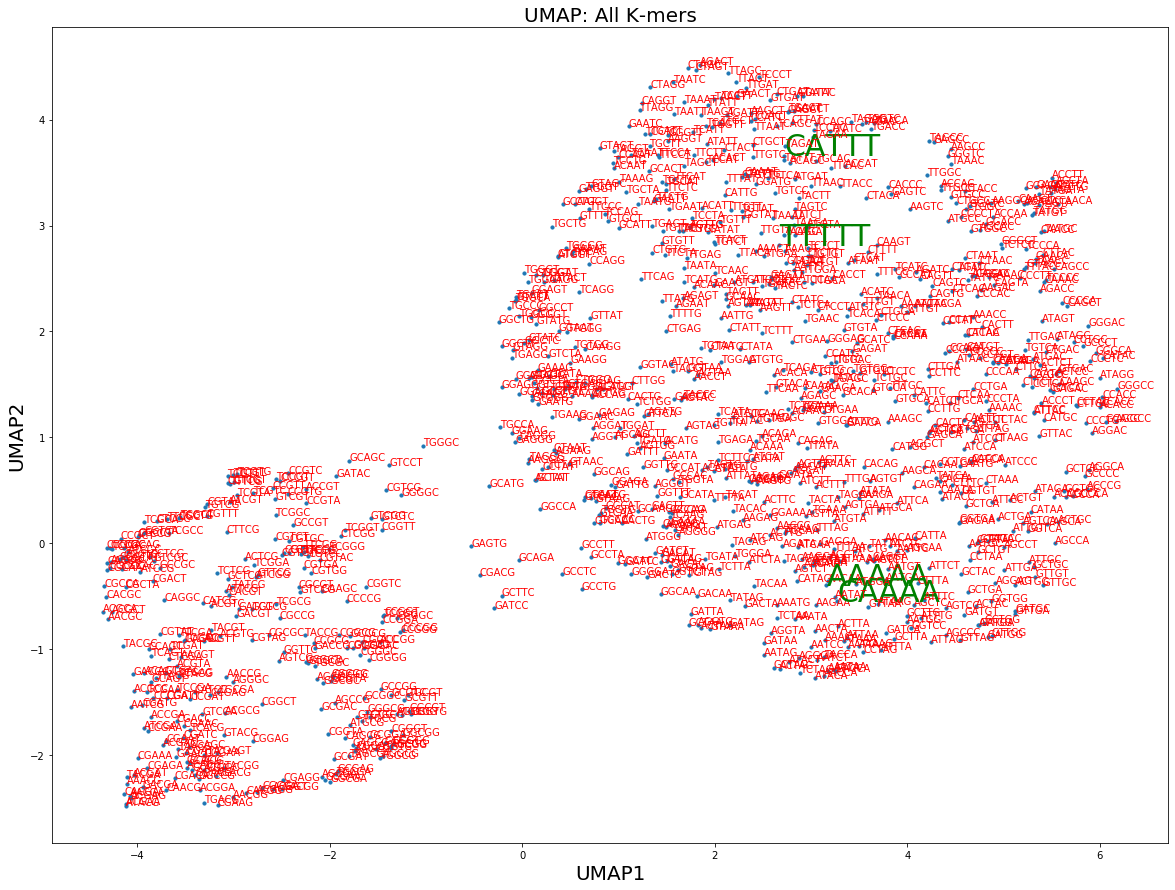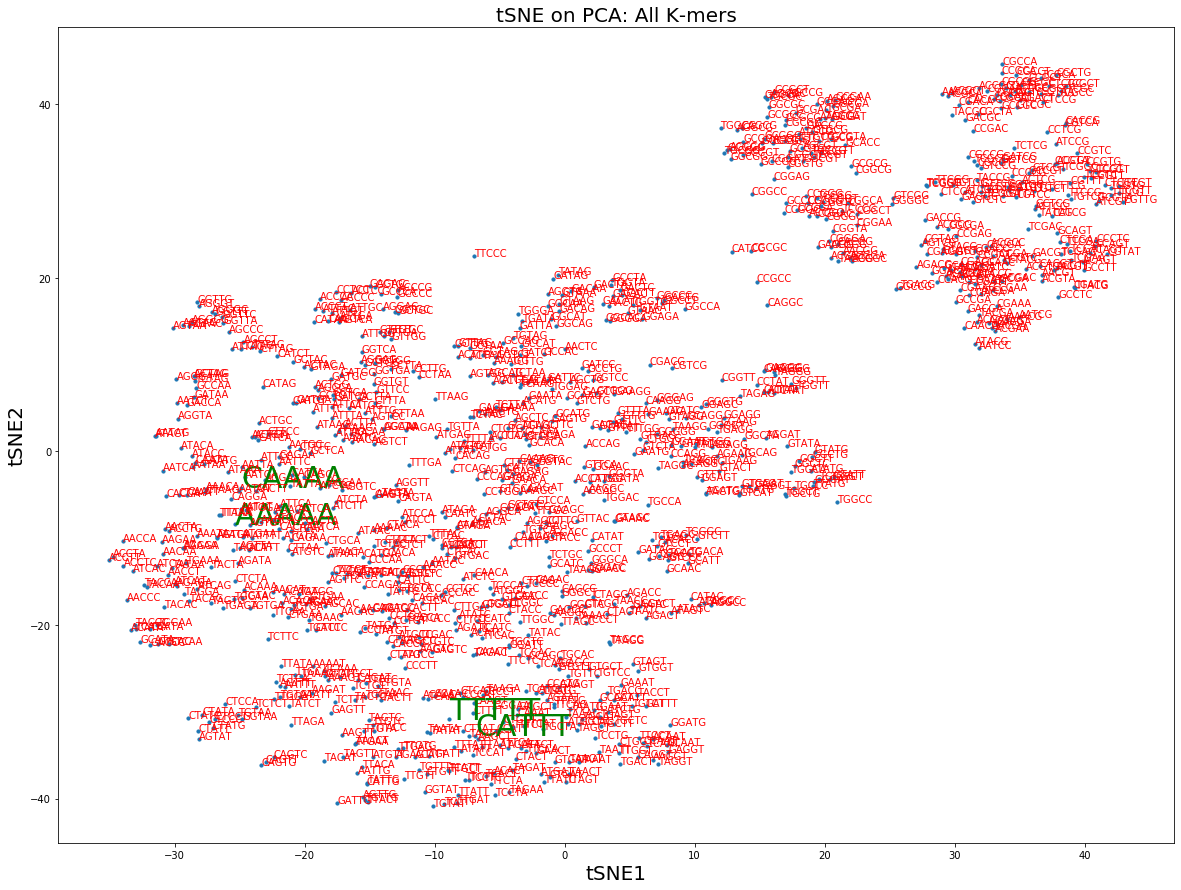I specifically highlighted by green the most predictive k-mers according to the Random Forest classifier that discriminates between Neanderthal introgressed vs. depleted sequences. As expected they all fall into the bigger AT-rich cluster. This Word Embedding visualization is yet another evidence of some structure present in the k-mer sentences, which is capable of predicting the Neanderthal ancestry and has something to do with the balance between GC- and AT-rich segments of DNA.

## Evolution Eliminates Neanderthal DNA from Genes

Summarizing everything discussed in the previous sections one can conclude that the presence of Neanderthal ancestry in our DNA suspiciously correlates with the GC / AT-content across the genome. It is well known that our genes are GC-rich, their GC-content is around 47% compared to 41% genome-wide, which is a dramatic difference in GC-content.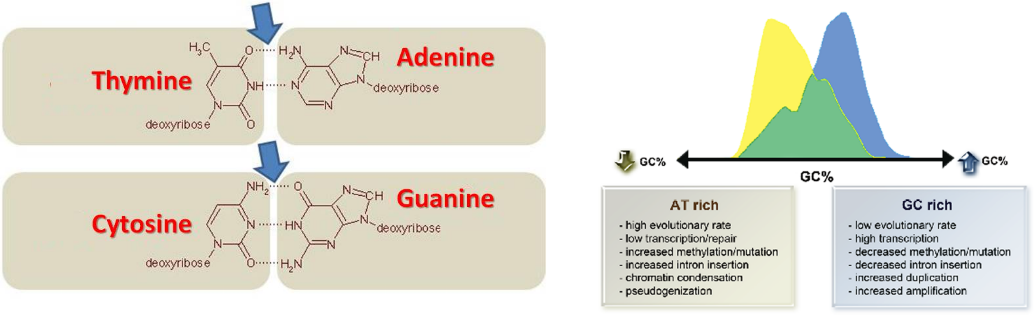Human genes are typically GC-rich which is indicative for absence of Neanderthal ancestry

Therefore we can ask how much actually the Neanderthal introgression and depletion regions overlap with the genes? We can quickly compute the number of intersects between the genes and introgression coordinates with bedtools intersect. However, since the coordinates of the Neanderthal depleted regions were previously selected randomly (we only demanded their non-overlapping with the introgression coordinates), we should repeat the selection procedure a number of times in order to estimate the significance of the intersects.

random_depl.py

``````import subprocess
import numpy as np
import seaborn as sns
import matplotlib.pyplot as plt

perm_n = []
for k in range(20):
chr_list = []; start_list = []; end_list = []
intr_lengths = list(intr_coords.iloc[:, 2] - intr_coords.iloc[:, 1])
a = 0
for i in range(intr_coords.shape):
chr_df = intr_coords[intr_coords.isin([intr_coords.iloc[i,0]])]
overlap = True
while overlap == True:
reg_start=
np.random.randint(1,int(chr_sizes[chr_sizes==intr_coords.iloc[i,0]].iloc[:,1]))
reg_end = reg_start + intr_lengths[i]
for j in range(chr_df.shape):
b1 = chr_df.iloc[j,1]; b2 = chr_df.iloc[j,2]
if (reg_start > b1 and reg_start < b2) or (reg_end > b1 and reg_end < b2) \
or (b1 > reg_start and b1 < reg_end) or (b2 > reg_start and b2 < reg_end):
overlap = True
break
else:
overlap = False
chr_list.append(intr_coords.iloc[i,0])
start_list.append(reg_start); end_list.append(reg_end)
a = a + 1
if a%20000 == 0:
print('Finished ' + str(a) + ' Neanderthal haplotypes')
depl_coords = pd.DataFrame({'0': chr_list, '1': start_list, '2': end_list})
depl_coords.to_csv("depl_temp.txt", index = False, header = False, sep = "\t")

with open('n_intersects.txt', 'w') as fp:
subprocess.run(['bedtools', 'intersect', '-a', 'depl_temp.txt', '-b',
'gene_coords.txt'], stdout = fp)
print(k, akey_n.shape)
print('**********************************************************')
perm_n.append(akey_n.shape)

plt.axvline(x = 140821, linewidth = 4, color = 'r')
sns.distplot(perm_n)
plt.title("Distribution of Gene-Depletion Intersects: Vernot and Akey, Science 2016",
fontsize = 30)
plt.xlabel("Number of Intersects Between Gene and Neanderthal Depleted Regions",
fontsize = 30)
plt.ylabel("Frequency", fontsize = 30)``````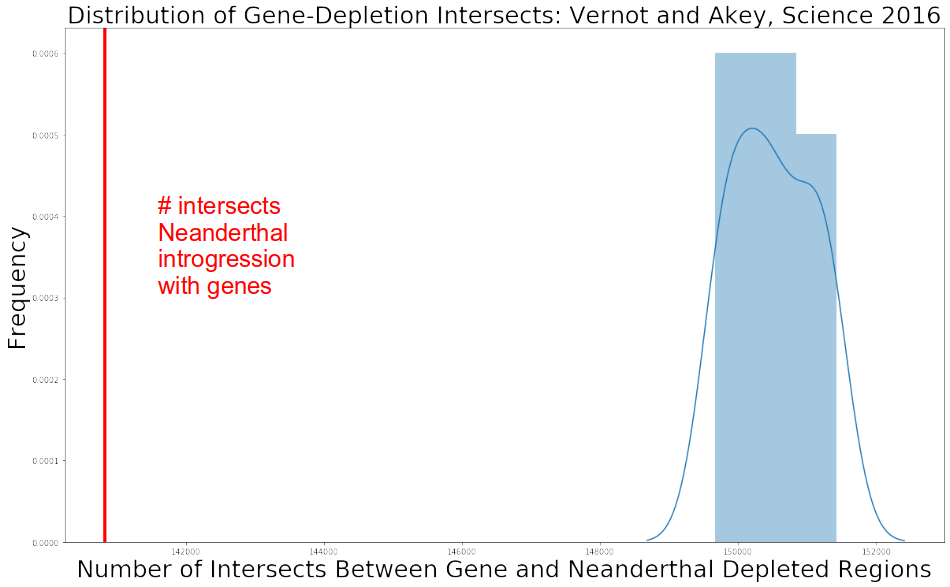We can see that the number of intersects between the genes and Neanderthal introgression segments, 140 821, is significantly lower than the number of intersects between genes and Neanderthal depleted regions. In fact, none of the 17 randomly drawn regions of Neanderthal depletion had the number of intersects with the genes below or equal 140 821, so we can compute p-value as p-value < 1 / 17. What we see is that the Neanderthal introgressed regions fall predominantly outside of the genes, which implies that Evolution did not prioritize the Neanderthal ancestry and tried to push it away from the most functional elements of our genome, which are protein-coding genes. Therefore for some mysterious reasons interbreeding with Neanderthals did not improve our fitness and Evolution tried to correct for this. They talk a lot in newspapers and magazines about a very cool fact of interbreeding between Modern Humans and Neanderthals, but they never mention that this was not beneficial for Modern Humans, do they?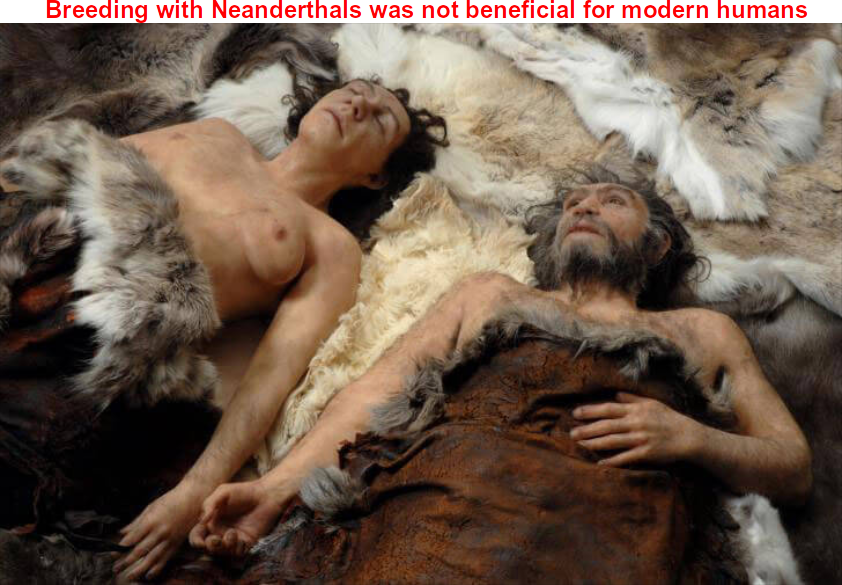## Summary

In this post, we have learnt about a huge potential of Machine / Deep Learning and Natural Language Processing (NLP) for Evolutionary Biology and Ancient DNA research areas, which is still not fully utilized. Genomics data provides one of the main sources of information in Evolutionary Biology and comprises millions and billions of short DNA fragments that can and should be analyzed with Deep Learning. Here we demonstrated how to prepare Genomics data for NLP and analyze it with Bag Of Words and Word Embedding. We trained a model capable of predicting whether a genomic sequence was inherited from Neanderthals. Using the model we constructed lists of genes that were likely inherited from Neanderthals and discovered a lack of archaic ancestry in our genes which suggests that interbreeding with Neanderthals was evolutionary, not beneficial for modern humans.

Important to mention that I am currently working on various types of neural network architectures with long memories across the sequence such as RNNs / LSTMs, CNNs / multichannel CNNs with application to Ancient Genomics and am going to come back to this topic in my later posts.

As usual, let me know in the comments your favorite area in Life Sciences which you would like to address within the Deep Learning framework. Follow me at Medium Nikolay Oskolkov, in Twitter @NikolayOskolkov and connect in Linkedin. The complete Jupyter notebook can be found on my github. I plan to write the next post about How to predict population size back in time with Deep Learning, stay tuned.

## Deep learning on graphs: successes, challenges, and next steps

Deep learning on graphs: successes, challenges, and next steps. TL;DR This is the first in a series of posts where I will discuss the evolution and future trends in the field of deep learning on graphs.

## Emojify - Create your own emoji with Deep Learning

Emojify - Create your own emoji with Deep Learning. We will classify human facial expressions to filter and map corresponding emojis or avatars.

## Batch Normalization: The Greatest Breakthrough in Deep Learning

It gets rid of the extreme gradients that accumulate, leading to the elimination of the major weight fluctuations that result from gradient build-up. This dramatically stabilizes learning.

## Few Shot Learning — A Case Study (2)

Implementations regarding all of above experiments alongside the different result plots are provided in GitHub repository.

## Paper Summary: Playing Atari with Deep Reinforcement Learning

This paper presents a deep reinforcement learning model that learns control policies directly from high-dimensional sensory inputs.